# How to Find the Highest Common Factor

How to Find the Highest Common Factor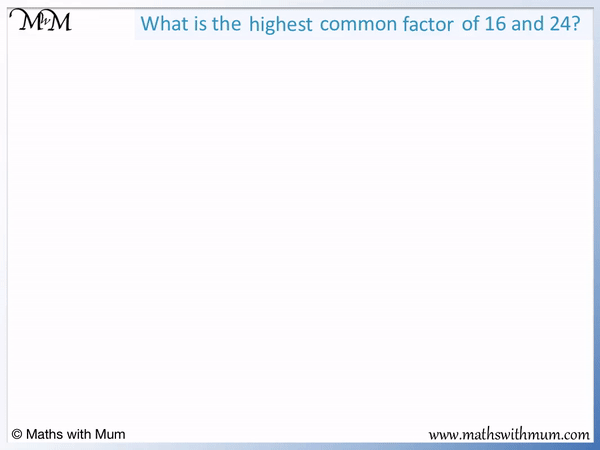• A factor is a number that divides exactly into a larger number.
• The highest common factor is the largest number that is a factor of two or more numbers.
• The highest common factor (HCF) is also called the greatest common factor (GCF) or greatest common divisor (GCD).
• To find the HCF, start by making a list of all of the factors for each number.
• The HCF is the largest number to appear in all of the lists.
• 8 is the largest number to appear in both lists, so 8 is the HCF of 16 and 24.

• Alternatively, find the HCF by listing the prime factors of each number.
• 16 = 2 × 2 × 2 × 2.
• 24 = 2 × 2 × 2 × 3.
• To find the HCF, multiply the numbers that are in common to both lists.
• 2 × 2 × 2 = 8 and so, 8 is the highest common factor of 16 and 24.

Make a list of all of the factors for each number.

The highest common factor is the largest number in common to all of the lists.• The highest common factor is the largest number that is in common to the list of factors of each number.
• The factors of 10 are: 1, 2, 5 and 10.
• The factors of 28 are: 1, 2, 4, 7, 14 and 28.
• The largest number that is in both lists is 2.
• The highest common factor of 10 and 28 is 2.

• Alternatively, we could find the HCF by listing prime factors.
• 10 = 2 × 5.
• 28 = 2 × 2 × 7.
• The single 2 is the only number in both lists of prime factors so 2 is the HCF.# Finding the Highest Common Factor

## What is the Highest Common Factor?

The highest common factor is the largest number that can divide exactly into all of the given numbers. For example, 5 is the highest common factor of 15 and 20. It is also known as the greatest common factor or greatest common divisor.

A factor is a number that divides into another number exactly. For example, 2 is a factor of 16.

Factors can often be written in pairs. For example, 2 × 8 = 16 and so, 2 and 8 are a factor pair for 16.

We will look at finding the highest common factor for 16 and 24.

The factors of 16 and 24 are shown below: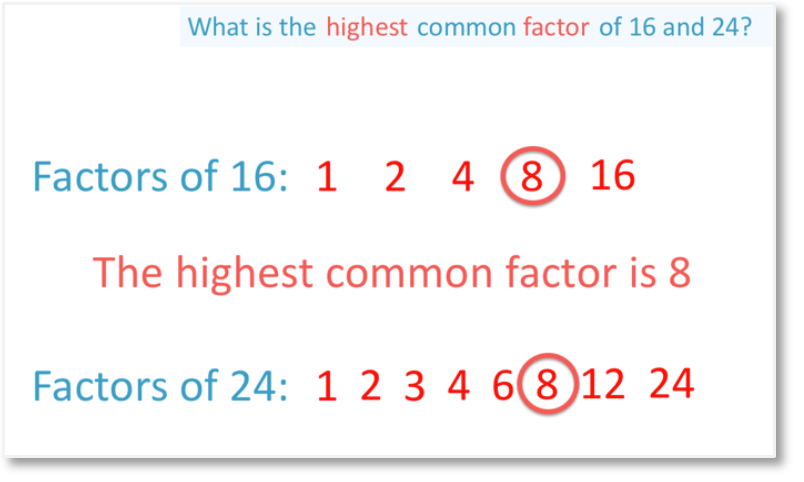All of the factors of 16 are: 1, 2, 4, 8 and 16.

All of the factors of 24 are: 1, 2, 3, 4, 6, 8, 12 and 24.The largest number in both lists is 8. Therefore the greatest common factor of 16 and 24 is 8. This means that 8 is the largest number that can divide exactly into both 16 and 24.

The greatest common factor is used in other branches of mathematics such as with fractions. For example, to simplify a fraction, divide the numerator and denominator by the greatest common factor of them both.

The greatest common factor is used to solve some real-life problems. For example, one cake is 20 cm long and another is 15 cm long. If both cakes need to be cut into smaller slices with equally sized lengths, what is the length of the largest slice of cake we can cut without wasting any cake?

We need to find the largest size slice that goes into both cake lengths. We need to find the largest number that divides into 15 and 20. This is the greatest common factor.

The greatest common factor of 15 and 20 is 5. So the cakes need to be cut into 5 cm slices so that all slices are the same size and no cake is wasted.

## How to Find the Highest Common Factor

To find the highest common factor, follow these steps:

1. List all of the factors of each number in separate lists.
2. The highest common factor is the largest number that is in common to all of the lists.
3. For example, we will find the highest common factor of 10 and 28.

The first step is to list all factors of each number.

The factors of 10 are 1, 2, 5 and 10.

The factors of 28 are 1, 2, 3, 4, 6, 8, 14 and 28.The final step is to find the largest number that is in both lists.

2 is the largest number that appears in both lists of factors and so, 2 is the highest common factor.

When teaching finding factors, it is important to remember that they often come in factor pairs. It is helpful to list the numbers in order and write down each pair at the same time.

Here is another example of finding the highest common factor of 2 numbers.

What is the highest common factor of 6 and 18?

The first step is to list all of the factors for both numbers.

The factors of 6 are 1, 2, 3 and 6.

The factors of 18 are 1, 2, 3, 6, 9 and 18.The next step is to find the greatest number that is in both lists of factors.

6 is the largest number in both lists and so, 6 is the highest common factor.In this example, the number 6 is the GCF despite it being one of the numbers itself.

If one of the numbers divides exactly into all of the other numbers, then this number is the greatest common factor. 6 is the greatest common factor of 6 and 18.

6 divides into both 6 and 18 exactly.

## How to Find the Greatest Common Factor by Prime Factorisation

To find the greatest common factor by prime factorization:

1. Find the prime factorisation of each number using a factor tree.
2. Find every number that is in common to each list of prime factors.
3. Multiply these numbers together to find the greatest common factor.

For example, find the greatest common factor of 16 and 20.

The first step is to find the prime factorisation of each number using a factor tree.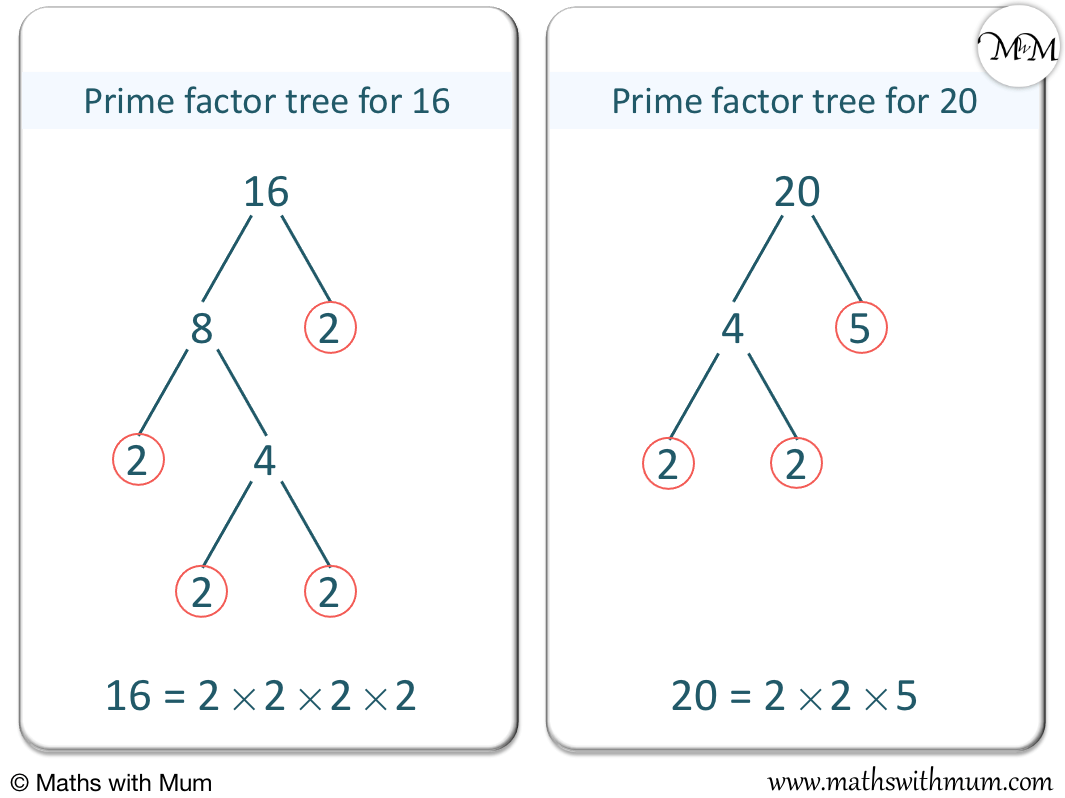16 = 2 × 2 × 2 × 2 and 20 = 2 × 2 × 5.

The next step is to find every number in common to each list of prime factors.

2 × 2 is in common to both lists.The final step is to multiply these common numbers together to find the greatest common factor.

2 × 2 = 4 and so, 4 is the GCF.

This means that 4 is the largest number that divides into both 16 and 20 exactly.

Here is another example of finding the highest common factor using prime factorisation.

What is the highest common factor of 27 and 36?

The first step is to find the prime factorisation of both numbers. The prime factorisation is the product of the circled prime numbers found in the prime factor tree.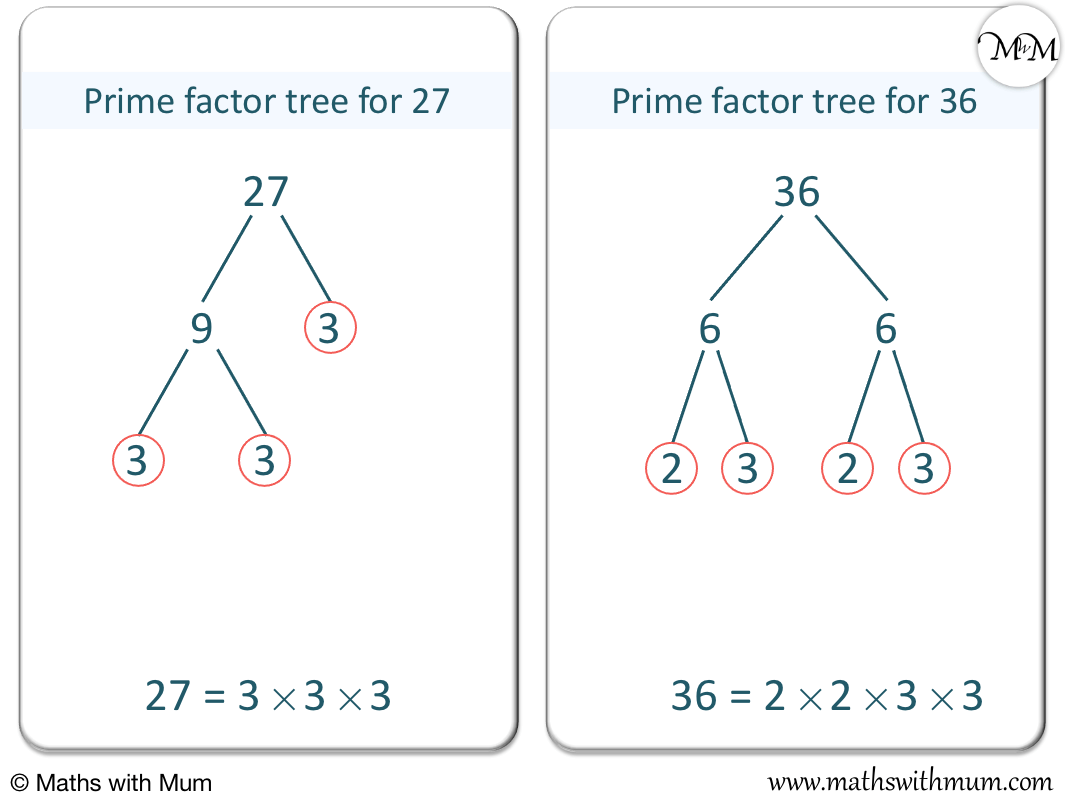27 can be written as 3 × 9. The 3 is prime and is circled. The 9 can be written as 3 × 3. Both of these threes can also be circled.

The number is written as the circled numbers on the prime factor tree multiplied together.

27 = 3 × 3 × 3.

36 can be written as 6 × 6. Each 6 can be written as 2 × 3. Both 2 and 3 are prime and so, can be circled.

36 = 2 × 2 × 3 × 3.

The next step is to find the numbers in common to both prime factor lists.

3 × 3 is in both lists. There are three 3’s multiplied together in 27 but there are only two 3’s multiplied together in 36. Therefore we only take 3 × 3 and not 3 × 3 × 3.

The final step is to multiply these numbers together to find the highest common factor.

3 × 3 = 9 and so, 9 is the HCF of 27 and 36. This means that 9 is the largest number that can divide exactly into both 27 and 36.

## Greatest Common Factor of 3 Numbers

To find the greatest common factor of 3 numbers, list all of the factors of each number in 3 separate lists. Then multiply all of the numbers that are in common to all 3 lists.

For example, find the greatest common factor of the 3 numbers of 12, 18 and 30.

This means to find the largest number that divides exactly into all three numbers.

There are two methods we can use to find the greatest common factor of three numbers. The first method is to find the largest number in common from their list of factors. The second method is to make a prime factorisation of each of the numbers and to multiply the numbers in common to each prime factorisation.

#### HCF of 3 Numbers: Method 1

The first step is to list all of the factors of each number.

The factors of 12 are 1, 2, 3, 4, 6, and 12.

The factors of 18 are 1, 2, 3, 6, 9 and 18.

The factors of 30 are 1, 2, 3, 5, 6, 10, 15 and 30.The next step is to find the largest number in common to all of the factor lists.

6 is the largest number in the factor lists of all three numbers and so, 6 is the greatest common factor.

This means that 6 is the largest number that divides exactly into 12, 18 and 30.

#### HCF of 3 Numbers: Method 2

The first step is to find the prime factorisation of each number using a prime factor tree.

12 can be written as 2 × 2 × 3.

18 can be written as 2 × 3 × 3.

30 can be written as 2 × 3 × 5.The next step is to find the numbers in common to all of the prime factorisation lists.

We have 2 × 3.

The final step is to multiply these numbers to find the highest common factor.

2 × 3 = 6 and so, 6 is the highest common factor of 12, 18 and 30.

When introducing the highest common factor, the easiest method to teach is the method of listing factors and looking for the largest number in each list. This is because you only need to know basic division to find factors.

Finding the highest common factor using prime factorisation is a more reliable method to teach with larger numbers but it requires the understanding of prime numbers and prime factor trees.Now try our lesson on How to Find the Lowest Common Multiple where we learn how to find the lowest common multiple.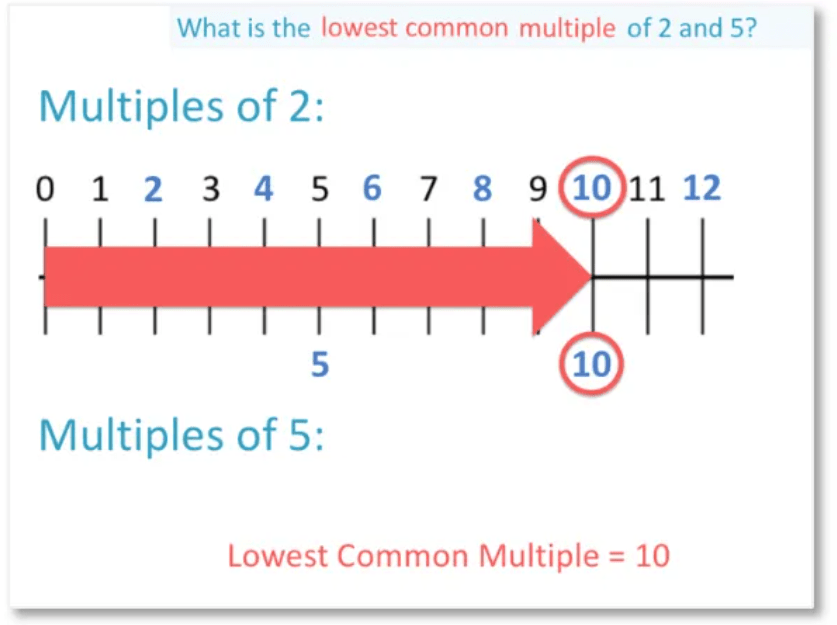error: Content is protected !!